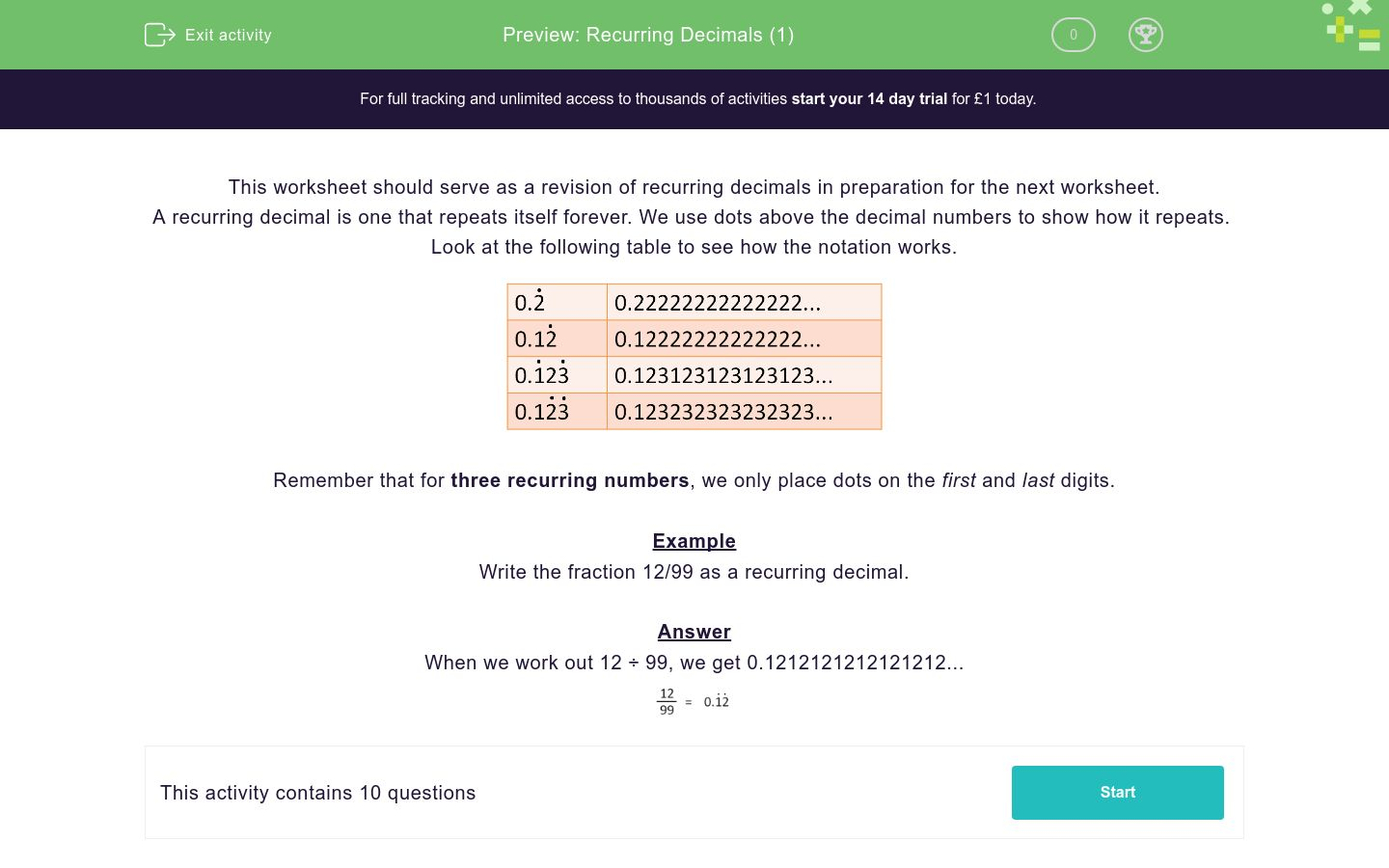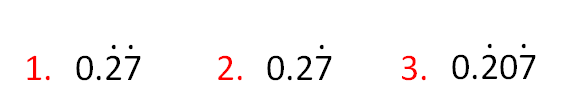# Recurring Decimals (1)

In this worksheet, students convert fractions to recurring decimals, using a calculator.Key stage:  KS 4

Curriculum topic:  Number

Curriculum subtopic:  Change Recurring Decimals into Their Corresponding Fractions and Vice Versa

Difficulty level:### QUESTION 1 of 10

This worksheet should serve as a revision of recurring decimals in preparation for the next worksheet.

A recurring decimal is one that repeats itself forever. We use dots above the decimal numbers to show how it repeats.  Look at the following table to see how the notation works.Remember that for three recurring numbers, we only place dots on the first and last digits.

Example

Write the fraction 12/99 as a recurring decimal.

When we work out 12 ÷ 99, we get 0.1212121212121212...Select the recurring decimal which is equal to:

 4 331.

2.

3.

Select the recurring decimal which is equal to:

 102 9991.

2.

3.

Select the recurring decimal which is equal to:

 34 3331.

2.

3.

Select the recurring decimal which is equal to:

 11 901.

2.

3.

Select the recurring decimal which is equal to:

 25 901.

2.

3.

Select the recurring decimal which is equal to:

 27 991.

2.

3.

Select the recurring decimal which is equal to:

 23 1111.

2.

3.

Select the recurring decimal which is equal to:

 15 371.

2.

3.

Select the recurring decimal which is equal to:

 6 111.

2.

3.

Select the recurring decimal which is equal to:

 127 2251.

2.

3.

• Question 1

Select the recurring decimal which is equal to:

 4 331.
EDDIE SAYS
4 ÷ 33 = 0.1212121212...
The 1 and the 2 recur.
• Question 2

Select the recurring decimal which is equal to:

 102 9993.
EDDIE SAYS
102 ÷ 999 = 0.102102102102...
The 1, 0 and 2 recur.
Dots go on the first and last digits only.
• Question 3

Select the recurring decimal which is equal to:

 34 3333.
EDDIE SAYS
34 ÷ 333 = 0.102102102102...
The 1, 0 and 2 recur.
Dots go on the first and last digits only.
• Question 4

Select the recurring decimal which is equal to:

 11 902.
EDDIE SAYS
11 ÷ 90 = 0.12222222222...
The 2 recurs.
A dot goes on the 2 but not on the 1.
• Question 5

Select the recurring decimal which is equal to:

 25 902.
EDDIE SAYS
25 ÷ 90 = 0.27777777777...
The 7 recurs.
A dot goes on the 7 but not on the 2.
• Question 6

Select the recurring decimal which is equal to:

 27 991.
EDDIE SAYS
27 ÷ 99 = 0.2727272727...
The 2 and the 7 recur.
Dots go on the 2 and the 7.
• Question 7

Select the recurring decimal which is equal to:

 23 1113.
EDDIE SAYS
23 ÷ 111 = 0.207207207207...
The 2, 0 and 7 recur.
Dots go on the first and last digits only.
• Question 8

Select the recurring decimal which is equal to:

 15 373.
EDDIE SAYS
15 ÷ 37 = 0.405405405405...
The 4, 0 and 5 recur.
Dots go on the first and last digits only.
• Question 9

Select the recurring decimal which is equal to:

 6 111.
EDDIE SAYS
6 ÷ 11 = 0.545454545454...
The 5 and 4 recur.
Dots go on both.
• Question 10

Select the recurring decimal which is equal to:

 127 2252.
EDDIE SAYS
127 ÷ 225 = 0.5644444444...
The 4 recurs.
A dot only goes on the 4 but not on the 5 or 6, because these digits don't recur.
---- OR ----

Sign up for a £1 trial so you can track and measure your child's progress on this activity.

### What is EdPlace?

We're your National Curriculum aligned online education content provider helping each child succeed in English, maths and science from year 1 to GCSE. With an EdPlace account you’ll be able to track and measure progress, helping each child achieve their best. We build confidence and attainment by personalising each child’s learning at a level that suits them.

Start your £1 trial